## Example Questions

### Example Question #1 : Probability & Statistics

A bakery sells two types of cookies: chocolate chip and snickerdoodles. Lucky customers randomly get a bonus brownie with their purchase according to the following probabilities: 10% of chocolate chip cookies come with a bonus brownie, and 25% of snickerdoodles come with a bonus brownie. If the bakery sells four times as many chocolate chip cookies as they sell snickerdoodle cookies, what is the percent likelihood that a sale chosen at random came with a bonus brownie?

10%

17.5%

20%

13%

13%

Explanation:

This probability problem involves the concept of expected value. If the bakery is four times as likely to sell a chocolate chip cookie as it is to sell a snickerdoodle, then you can say that chocolate chip cookies are ⅘ of the total number of cookies sold, and that snickerdoodles are ⅕ of the total cookies sold.

This means that ⅘ of the cookies carry a 10% probability of a bonus brownie, and ⅕ of the cookies carry a 25% probability. You can then turn this into an equation:

⅘ (10%) + ⅕ (25%) is the expected probability of a random cookie winning the brownie. That means that the answer is 8% + 5% = 13%.

### Example Question #1 : Probability & Statistics

Emily keeps 12 different pairs of shoes (24 individual shoes in total) under her bed. If her dog drags out two shoes at random, what is the probability that he drags out a matching pair of shoes?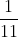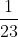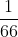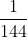Explanation:

Whenever you're dealing with "pairs probability" it is important to ask yourself whether your job is to calculate the probability of a specific pair (e.g. a pair of red shoes) or of just "any" pair whatsoever. Here the question asks for "a pair" and not a specific pair, so it is important to recognize that the first shoe the dog pulls out can be any shoe, but the second shoe he pulls must match the first. So the only pull that "matters" is the second one - on that draw he must match the one he drew first.

At that point there are 23 shoes left (everything but the one he picked first), and only one that will match the first one (since we're dealing with 12 different pairs). This means that the probability is.

### Example Question #2 : Probability & Statistics

A jar contains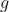green marbles and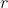red marbles. If 2 green and 14 red marbles are added, which of the following expresses the probability of then selecting a green marble at random?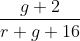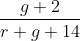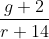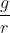Explanation:

The probability of selecting a green marble at random is, conceptually, the number of green marbles divided by the number of total marbles. Since 2 green marbles were added to the original total ofgreen marbles, the number of green marbles to select is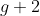. And the total number of marbles is calculated as the starting total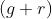plus the 2 green and 14 red marbles added, making the new total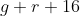. Therefore, the correct answer is the new number of greens plus the new number total:.

### Example Question #1 : Probability & Statistics

A jar contains 6 chocolate candies and 4 mint candies. If Ben reaches into the jar to draw and eat two candies at random, what is the probability that he eats two chocolate candies?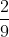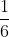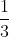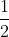Explanation:

This problem involves two critical elements of probability: dependent probability (when the outcome of one event then impacts the probability of the next event, because there are now fewer items to choose from) and sequential probability (when you're asked for the probability of multiple events happening in sequence, like "chocolate, then chocolate" here).

To start, note that the probability of drawing a chocolate candy on the first draw is calculated by taking the 6 chocolate candies and dividing by the 10 total candies, so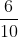.

For the second draw, there will only be 9 candies left (he will have already drawn one), and in theof outcomes in which he has already chosen chocolate, there will be 5 chocolate candies left. So that probability is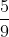.

When you need a particular sequence of events to happen (chocolate, then chocolate) you multiply those probabilities together to find the probability of that sequence. That would mean that the probability is then: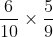The answer, therefore, is.

### Example Question #1 : Probability & Statistics

A basketball team’s current roster consists ofguards and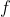forwards. If 2 guards join and 1 forward leaves, what is the probability that a player chosen at random is a guard?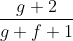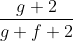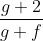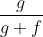Explanation:

To find the chances of picking a guard, our formula should be the number of guards divided by the total number of players. We start withguards andforwards, and then add 2 guards and subtract one forward. So after the roster changes, we haveguards, and we have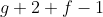total players. Since the probability of an outcome is the number of options that give you that outcome (guards) divided by the number of total options (all players), our probability is then.

### Example Question #1 : Calculating Probability

A jar contains 6 red marbles and 9 blue marbles. If Evelyn reaches into the jar and simultaneously draws two marbles at random, what is the probability that she will draw two marbles of the same color?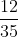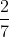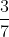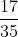Explanation:

With this probability problem, it is important to note that there are two paths to the desired outcome: two red marbles, and two blue marbles. So you will want to calculate the probability of each path and then add them together.

To draw two red marbles, Evelyn would need to draw one of 6 red marbles from the jar of 15, and then draw one of the remaining 5 red marbles from the remaining 14. So that probability looks like: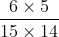This fraction reduces to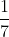.

To draw two blue marbles, Evelyn would need to draw one of the 9 blues from the jar of 15, and then one of the remaining 8 blues from the remaining 14. That probability looks like: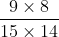This fraction reduces to.

When you add the two probabilities together (adding because red, red and blue, blue are two distinct probabilities that each satisfy the question), you'll get.

### Example Question #6 : Calculating Probability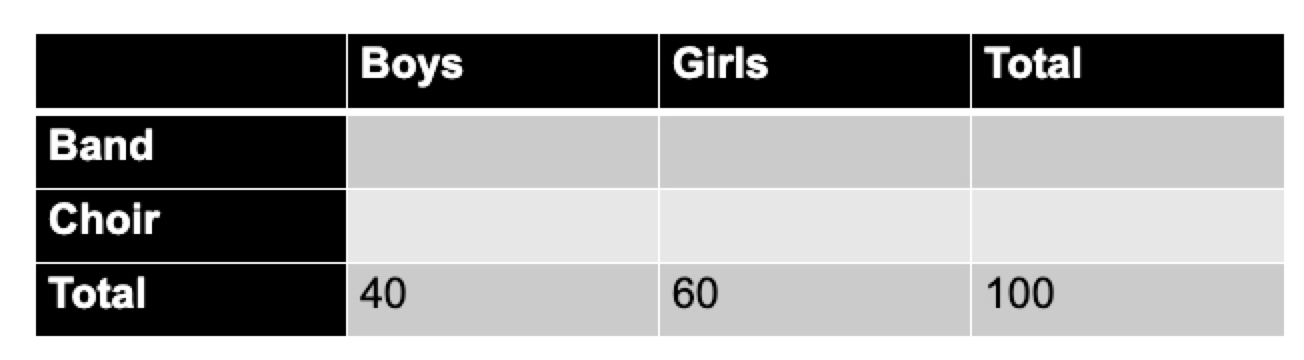The incomplete table above summarizes the breakdown of 100 students sent from Buena High School to perform in a holiday parade.  Of the girls sent to perform, twice as many are in the band as in the choir.  Of the choir members sent to perform, there are twice as many girls as boys.  If one of Buena High School’s student musicians is selected at random, what is the probability that it is a boy from the band?

10%

40%

20%

30%

30%

Explanation:

This probability problem begins with an incomplete table, but provides you with the information necessary to fill it in. You know that there are 60 total girls, and that twice as many girls are in the band as the choir.  So you can set up a system of equations for the girls:

B + C = 60

B = 2C

So 2C + C = 60, meaning that, among the girls column, C = 20 and B = 40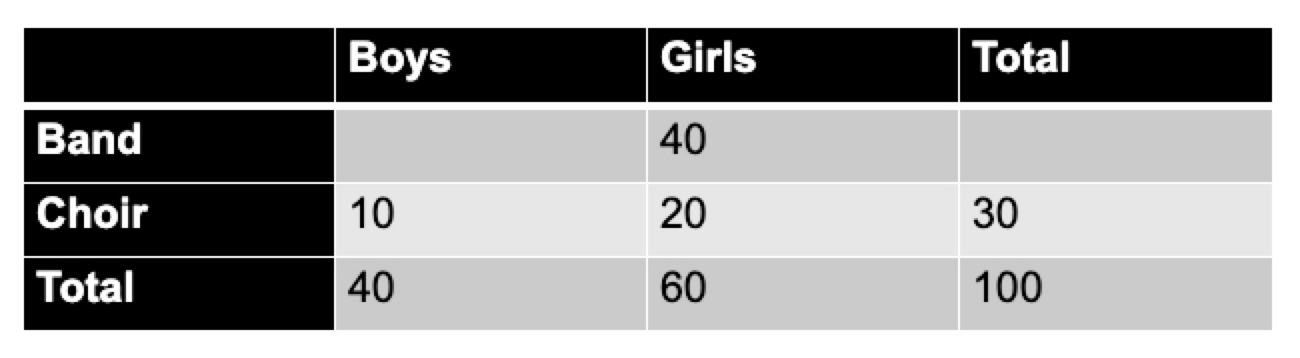You then know that in the choir, there are twice as many girls as boys. Since there are 20 girls in the choir, you know that there are 10 boys in the choir. When you fill that into the table, you can then see that there must be 30 total choir members: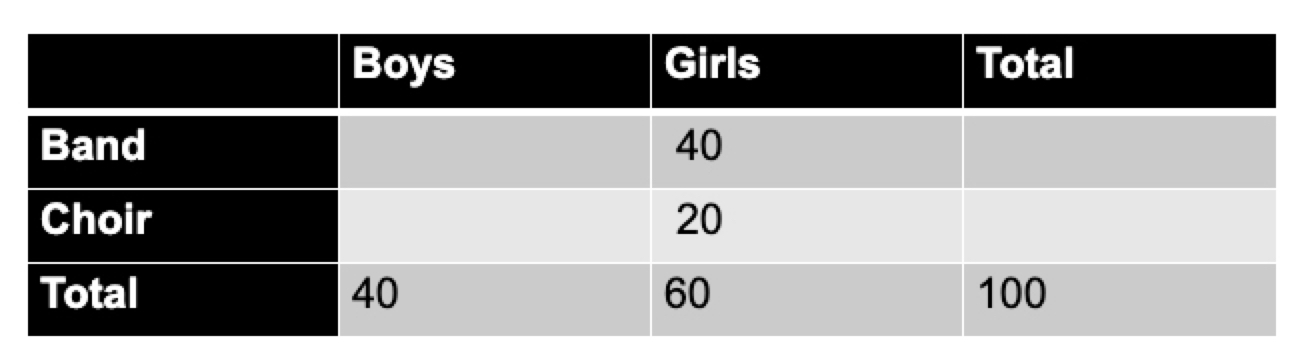And you can then fill in the rest of the table. With 40 total boys and 10 in the choir, there must be 30 boys in the band, and that then means that, in the band, 30 boys + 40 girls equals 70 total members.

The question asks for the probability that any one student at random is a boy in the band. There are 30 such boy band members out of 100 total students, so the answer is 30%.

### Example Question #8 : Calculating Probability

When throwing darts, Emma hits the bullseye target 10% of the time, lands in an even-numbered zone 30% of the time, lands in an odd-numbered zone 30% of the time, hits the unscored outer edge of the board 10% of the time, and misses the board completely 20% of the time. Which of the following reflects the probability that one of Emma's darts that does not miss the board completely is a dart that hits the bullseye target?

10%

17.5%

12.5%

15%

12.5%

Explanation:

Remember that the probability of an outcome occurring is calculated as the number of favorable outcomes (in this case, bullseye targets) divided by the number of total outcomes. And here we know that the number of total outcomes in the provided situation has "changed" from the provided probabilities. If Emma were to throw 100 darts, 10 would hit the bullseye, 30 would land on an odd number, 30 would land on an even number, 10 would hit the unscored outer edge of the board, and 20 would miss completely. But since we're told that she did not miss the board completely, our calculation is that the number of favorable outcomes is still 10, but the number of total outcomes omits those 20 total misses. So the total number of outcomes is 10 bullseyes + 30 odds + 30 evens + 10 outer edges = 80.

Therefore the probability is 10 favorable outcomes divided by 80 total outcomes, for a probability of 12.5%.

### Example Question #9 : Calculating Probability

A mountain resort will hold its annual one-day snowboarding competition if it snows on either Saturday or Sunday, but if it does not snow at all it will not hold the event. If there is a 70% chance that it snows on any given day at the resort, what is the probability that the event will be held?

70%

91%

90%

81%

91%

Explanation:

In approaching this conditional probability problem it is important to recognize that you do not need it to snow BOTH days in order for the event to run: as long as it snows on one or the other the event will be held. So this problem is asking for the probability of snow on either Saturday or Sunday. There are a few ways to determine this probability.

One is to note that if it snows Saturday (70%), then the event will run, but that if it does NOT snow Saturday (30%) then there's a 70% probability that it will still snow Sunday. So the two sequences of "Snows Saturday" or "Doesn't Snow Saturday, but then Snows Sunday" will combine for the total probability of the event running:

Snows Saturday = 70%
Does Not Snow Saturday, then Snows Sunday = (30%)(70%) = 21%

Snows either Saturday or Sunday = 70% + 21% = 91%.

You could also note that the only outcome that does NOT work for you is if it fails to snow both days. Everything else does work, so you could subtract the probability of "No Snow, then No Snow" from 100% to get to the total:

100% - Neither
100% - (30%)(30%) = 100% - 9% = 91%.

### Example Question #541 : Sat Math

Jenny's cabinet contains 4 bags of tortilla chips, 4 bags of potato chips, and 5 bags of popcorn. Jenny reaches into the cabinet and draws a bag at random, places it on the counter, and returns to draw one more bag at random. What is the probability that she pulls out two bags of popcorn?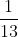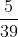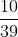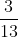Explanation:

With dependent probability - probability for which the outcome of one event impacts the probability of the next event - it is important to consider the sequence of events. Here, Jenny needs a particular sequence: Popcorn, then another Popcorn.

For her first selection, there are 5 bags of popcorn out of 13 total bags, so the probability is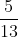.

For her second draw, there will be 4 bags of popcorn left out of a total of the 12 remaining bags, for a probability of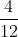, which reduces nicely to.

Because she needs a bag of popcorn AND another bag of popcorn, you'll multiply the two individual probabilities. When you multiply the two fractions, you have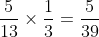.

### All SAT Mathematics Resources